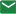Home / Product / Steam Activity / Mathematics Toy Kit

If you are interested in our products or have any questions, please consult us.Contact us

## INDUSTRY KNOWLEDGE EXTENSION

Mathematics Toy Kits offer numerous benefits for children's enlightenment and mathematical development. Here are some key advantages:
Conceptual Understanding: Mathematics Toy Kits provide hands-on experiences that help children develop a concrete understanding of mathematical concepts. Through manipulatives, such as number blocks, geometric shapes, or counting objects, children can visually and physically engage with abstract mathematical ideas, making them more tangible and comprehensible.
Problem-Solving Skills: Mathematics Toy Kits often include puzzles, games, and activities that require problem-solving skills. Children are challenged to think critically, analyze patterns, apply logical reasoning, and develop strategies to solve mathematical problems. These experiences foster the development of problem-solving skills that can be applied beyond mathematics.
Numeracy Skills: Math Toy Kits help children develop numeracy skills, including counting, number recognition, and basic arithmetic operations. Manipulating objects or using visual representations, such as number lines or ten frames, helps children grasp number concepts and build a strong foundation for further mathematical learning.
Spatial Reasoning: Some Mathematics Toy Kits involve geometric shapes, tangrams, or spatial puzzles. These activities enhance children's spatial reasoning skills, which are essential for understanding spatial relationships, geometry, and visualization. Spatial reasoning also has connections to skills in STEM fields and problem-solving abilities.
Logical Thinking and Reasoning: Mathematics Toy Kits encourage logical thinking and reasoning skills. Children learn to make connections between different mathematical concepts, recognize patterns, and apply deductive and inductive reasoning. These skills are valuable not only in mathematics but also in various aspects of life, such as critical thinking and decision-making.
Mathematical Communication: Mathematics Toy Kits often promote mathematical communication and language development. Children can engage in discussions about mathematical concepts, explain their thinking, and articulate their reasoning. This enhances their communication skills, fosters the ability to express ideas mathematically, and strengthens their overall language development.
Mathematical Confidence: Engaging with Mathematics Toy Kits in a fun and interactive way helps children build confidence in their mathematical abilities. As they successfully solve problems and explore mathematical concepts, they develop a positive attitude towards mathematics and gain the confidence to approach more challenging mathematical tasks.
Mathematical Creativity: Mathematics Toy Kits can spark mathematical creativity by encouraging children to explore different approaches, find multiple solutions, and create their own mathematical models or patterns. This nurtures their creativity while developing their mathematical thinking.
Real-World Application: Mathematics Toy Kits often provide opportunities for children to apply mathematical concepts to real-world situations. They can engage in measurement, money concepts, time, or other practical applications, making mathematics relevant and meaningful to their daily lives.
Mathematics Toy Kits provide a hands-on and engaging approach to learning mathematics. They foster conceptual understanding, problem-solving skills, logical thinking, and mathematical confidence, laying a strong foundation for children's mathematical enlightenment and future success in the subject.